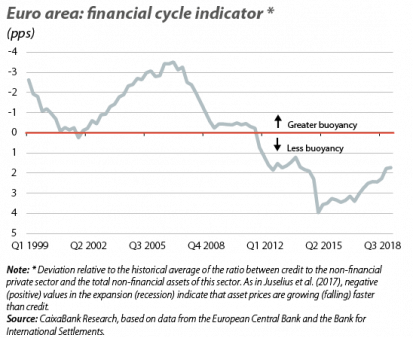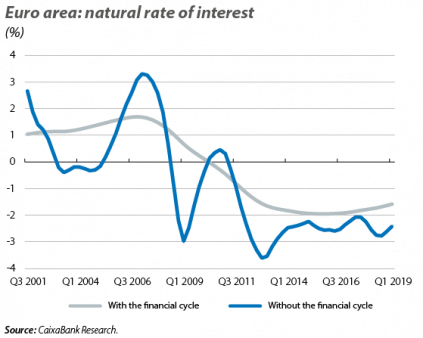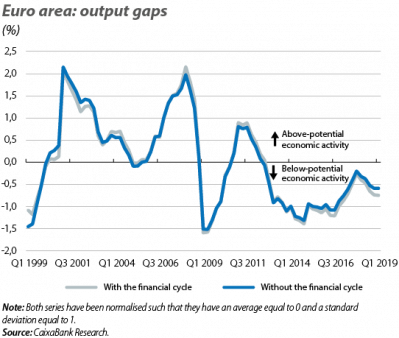# The financial cycle and the era of low interest rates: a change of narrative?

Content available in
Gabriel Ramos
Pablo Pastor y Camarasa
March 17th, 2020

The financial cycle plays a key role in the functioning of the economy, as we have seen in the previous articles of this Dossier. But what are the specific consequences of the relationship between the financial and business cycle? Below we analyse its implications for one of the key macrofinancial relationships: the one that exists between the financial cycle and equilibrium interest rates.

There is a broad consensus among economists that underlying transformations, such as the ageing of the population or the loss of buoyancy in productivity, have led to a drop in equilibrium, or natural, rates of interest.1 According to this consensus, to the extent that this decline is structural, monetary policy must adapt and, in times of economic weakness, use new tools to relax financial conditions (such as asset purchases or forward guidance). However, this view has been built and has gained prominence without taking into account the role of the financial cycle. Below, inspired by the analysis that Juselius et al. (2017)2 performed for the US, we study how the narrative of «the era of low interest rates» in the euro area changes when the financial cycle is incorporated into the equation.

The dominant narrative and its missing piece: the financial cycle

The natural rate of interest is the key benchmark for setting either accommodative (rates below the natural rate) or restrictive (above the natural rate) monetary policy. The difficulty for central banks lies in the fact that this rate is not observable. However, it is possible to infer it if we examine the economy carefully: when economic growth is accompanied by a rise in inflation, price pressures suggest that the economy is growing above its ability or its potential. From this situation, we can deduce that monetary policy is too lax, that is, the interest rate is below the natural rate (and vice versa). This simple relationship between economic activity, inflation and interest rates drives the estimates that show a widespread and sustained decline in natural rates of interest in the major advanced economies.3 But what if this relationship is incorrect (for example, it may give too much weight to the link between inflation and economic activity) or incomplete (it omits relevant dynamics)?

In the years leading up to the Great Recession, the economic activity of the euro area was highly buoyant. Under the prism of stable inflation close to 2%, this should have been sustainable. In retrospect, we know that this was not the case, but rather that the growth was fuelled by a significant expansion of credit. Also, in recent years there has been a weakening of the link between economic activity and inflation. This suggests that, at present, price trends give us less information about the sustainability of growth. Therefore, these experiences advise us to supplement the economic activity-inflation-interest rate relationship with an analysis of the state of the financial cycle. After all, if we do not incorporate the financial cycle (it is not normally not incorporated into the most widely-known estimates of the natural rate), then our only source of information for the sustainability of growth would be inflation. Moreover, we would attribute fluctuations in economic activity solely to changes in monetary policy.

Equilibrium interest rates and the financial cycle: an empirical incursion

To discover how the estimate of the natural rate of interest of the euro area changes with the incorporation of the financial cycle, we must include this cycle in the inflation-activity-interest rate trinomial. As we have seen previously, the classic view of the trinomial has two parts to it: the relationship between inflation and activity, on the one hand, and the link between activity and interest rates on the other. In order to incorporate the financial cycle into the analysis we will keep the first part intact and add it to the second part.4 In other words, there will be two possible explanations for deviations in economic activity with respect to its potential: how accommodative or restrictive monetary policy is (as determined by the difference between the real observed interest rate and the natural rate) and the state of the financial cycle.

How do we measure the financial cycle of the euro area? Given that we reproduced the estimates of the natural rate of interest of the US according to Juselius et al. (2017), we will rely on the same indicator used by these authors: non-financial private sector leverage.5 As shown in the first chart, this indicator plots a reasonable cycle for the euro area: the financial cycle experienced a phase of expansion in 2002-2008, followed by a correction after the financial and sovereign debt crisis, while in recent years it has recovered thanks to the consolidation of the economic recovery.

How does the story change?

Our revised estimate of the natural rate of interest for the euro area (see the technical details in footnote 4) shows the importance of the financial cycle. In fact, the message is similar to that offered by Juselius et al. (2017) for the US: the natural rate of interest continues to show a downward trend when we factor in the financial cycle (confirming the consensus view among economists), but its decline is less significant and more gradual (see second chart).6

To understand how the estimate of the natural rate changes with and without the financial cycle, we need to take a step back and observe something which, at first glance, might seem contradictory: in the third chart we see that the output gap of the euro area (i.e. the difference between the observed growth and its potential rate) which we estimate in both cases is very similar. However, in reality this finding is not unexpected: the financial cycle does not play a direct role in the inflation-activity relationship, given that we have not altered the first part of the trinomial.7 The big difference is found in the factors that drive the output gap: if we ignore the financial cycle, its fluctuations should be fully transmitted to the natural rate (hence it is more volatile when we ignore it). As an example, if we ignore the financial cycle, the double recession in the euro area (2008-2009 and 2011-2013) translates into a significant and double fall in the natural rate. In contrast, when we take the financial cycle into account, we see that it explains a significant part of the two recessions and that the decline in the natural rate over the course of the crisis is much more gradual.

In addition, there are two other interesting periods during which, when we consider the financial cycle, we discover that the natural rate of interest was higher than it appeared. On the one hand, in the years 2002-2005, not only did economic activity experience a boom (which in itself suggests an accommodative monetary policy), but so did the financial cycle. This indicates that monetary policy was even more accommodative than we thought (this is reflected in a higher natural rate when we take the financial cycle into consideration). On the other hand, in recent years, the financial cycle is still in a recovery phase. This is why we observe a higher natural rate of interest in this period, which indicates that monetary policy has been more expansionary, and this has compensated for the lower momentum of the financial cycle.

In short, the financial cycle helps us have a richer and more accurate understanding of the behaviour of the natural rate of interest. Although the natural rate is showing a downward trend in both the US and the euro area, we see significant differences in their level, which raises the question about the extent to which the central banks have properly calibrated their reference interest rates. This invites us to reflect on the direction of monetary policy, a subject to which we dedicate the next article of this Dossier.

Javier Garcia-Arenas, Gabriel Lobato Ramos,

Adrià Morron Salmeron and Pablo Pastor y Camarasa

1. The natural rate of interest is that which balances the supply and demand of savings and allows economic activity to grow in accordance with the economy’s potential and with stable inflation. See the article «The widespread fall in interest rates: a global trend» in this same Dossier. In addition, we present a complete analysis of the natural rate and the causes of its decline in the Dossier «The future of financial conditions: a paradigm shift?» in the MR02/2019.

2. M. Juselius et al. (2017). «Monetary Policy, the Financial Cycle, and Ultra-Low Interest Rates». International Journal of Central Banking. We would like to thank Mikael Juselius for sharing with us a version of the code with which they developed the econometric analysis.

3. Such as that of Holston et al. (2017). «Measuring the Natural Rate of Interest: International Trends and Determinants». Journal of International Economics 108, supplement 1 (May): S39–S75.

4. Analytically, we represent inflation, the output gap and the financial cycle with $$\pi$$, $$\widetilde\gamma$$, $$\widetilde{lev}$$ and we infer the natural rate $$r^\ast$$ from the estimate of the following system of equations, using data for the euro area of the period between 1999 and the present:

$$\pi_t\;-\;\pi^\ast\;=\;\alpha_{\pi\;}\;\left(\pi_{t-1}\;-\;\pi^\ast\right)\;+\;\alpha_\gamma\;{\widetilde\gamma}_t\;+\;\varepsilon_{\pi t}\\{\widetilde\gamma}_t\;=\;\beta_\gamma\;{{\widetilde\gamma}_{t-1}}_{}\;-\beta_{r\;}\left(r_{t\;}-r^\ast\right)\;-\;\beta_{lev}\;{\widetilde{lev}}_t\;+\;\varepsilon_{\gamma t}\\{\widetilde{lev}}_t\;\;=\;\delta_{lev}\;{\widetilde{lev}}_{t-1}\;+\delta_{r\;}\left(r_{t\;}-r^\ast\right)\;+\;\delta_d\;{\widetilde{dsr}}_{t-1}\;+\;\varepsilon_{lt}$$

The first equation allows us to infer the output gap based on the observed inflation, while the second allows us to infer the natural rate of interest based on the output gap and the observed financial cycle. i.e. We reproduce the exercise that Juselius et al. (2017) carried out for the US, but using data for the euro area. The full description of the statistical methodology can be found in their article, which sets out the complete and somewhat more extensive system (for example, it expands on the role of the debt service ratio, $$\widetilde{dsr}$$, which we do not set out here). To estimate $$r^\ast$$ in the absence of the financial cycle, we apply $$\beta_{lev}\;=\;0$$ and ignore the third equation.

5. Specifically, we use the ratio between credit to the non-financial private sector and the value of the non-financial assets of this sector (in its deviation relative to the historical average).

6. The natural rates of interest presented in this article for the euro area differ from those of Holston et al. (2017) set out in the article «The widespread fall in interest rates: a global trend» of this Dossier. This reflects small methodological differences between the two methods and illustrates the uncertainty surrounding these estimates, an aspect which we discuss in more depth in the last article of this Dossier, «The uncertainty surrounding the natural rate of interest».

7. The informative role of inflation may have changed when including the financial cycle if the new estimates indicated very different values for $$\alpha_\gamma$$. However, in our estimates, $$\alpha_\gamma$$ does not substantially change.

Gabriel Ramos
Pablo Pastor y CamarasaLong-term trends

## Macrofinance

What factors will determine the evolution of interest rates, investment sentiment and macro-financial conditions in general?